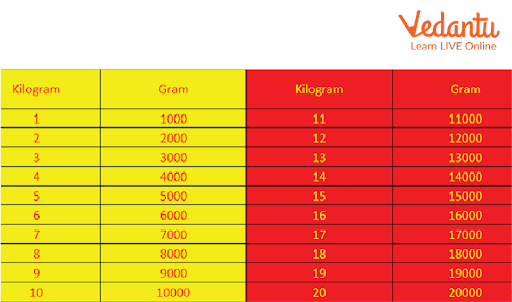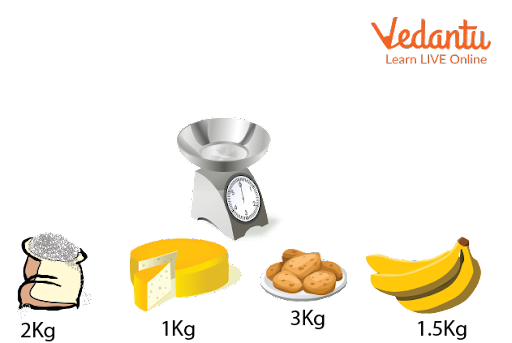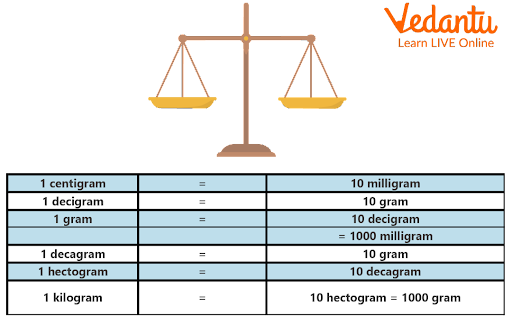Courses
Courses for Kids
Free study material
Offline Centres
More

# Kilogram ChartLast updated date: 04th Dec 2023
Total views: 103.2k
Views today: 3.03k## Introduction to Kilograms

As we all know, a kilogram is a standard unit of mass or weight. The international system of units will consider the kilogram as the SI unit of mass or weight. The word kilogram is a combination of "kilo" and "gram." These are Greek words. The word "kilo" means thousands, and the word "gram" refers to grammar; it means a small unit or weight. The kilogram is represented by the "kg” standard unit of mass or weight.

### Kilogram Chart:

The kilogram, denoted by the unit sign kg, is the unit of mass in the International System of Units (SI). It is a unit of measurement that is widely used in research, engineering, and commerce across the globe. Its meaning is "1,000 gram."

Here are some other measurements related to Kilogram.

1 Kg = 2.205 pounds

1 kg = 35.274 ounces

1 kg = 0.001 tonnes

1 kg = 1000 gram

1 kg = 100000 milligram

Gram Milligram Kilogram Chart:

Here we will define the relationship between kilogram gram and milligram through this given chart.

 Milligram Gram Kilogram 1mg 0.001gm 0.000001kg 1000mg 1gm 0.001kg 1000000mg 1000gm 1kg

Gram Kilogram Chart:

Here we want to define the relation between kilogram and gram. As we all know, the kilogram is the bigger unit and a gram is the smaller unit of mass. In other words, we can say that the thousandth part of a gram is equal to a kilogram quantity of any object.Gram Kilogram Chart

### Milligram Gram Kilogram Chart:

In this, we will cover the conversion from a milligram to a kilogram of a few weights. This will help to understand the conversion in simple terms.

Milligram

Gram

Kilogram

5000

5

0.005

10000

10

0.001

2000000

2000

2

18000000

18000

18

70

0.007

0.000007

100

0.0100

0.0001

### Gram and Kilogram Chart:

As we know that gram and kilogram are both units of weight or mass. Gram is used for smaller objects measurement and kilogram is determined as the larger or heavier object for measurement. In upcoming examples, we will understand the real-life examples of grams and kilograms.Kilograms and Grams Examples

In the above example we can see that the banana is 1 kg and 500 grams, the rice is 2 kg, and cheese is 1 kg. These are the examples that we use in our day-to-day life.Gram and Kilogram Chart

### Kilogram Measurement Chart:

As we all know that kilogram is the standard unit of weight. A kilogram is equal to 1000 grams of an object.

1 Kilogram = 1000 grams

We can measure kilogram measurement in different terms. We will use different types of measurement apparatus to measure the actual weight of any object. We can use different types of weight bats like 50 grams, 100 grams, 250 grams, 500 grams, 1 kg, 2 kg, 5 kg, and many more.

Kilogram Conversion Chart:

Conversion Chart;

1 milligram = 0.001 gram

1 centigram = 0.01 gram

1 decigram = 0.1 gram

1 kilogram = 1000 grams

1 gram = 1000 milligramsKilogram Weighing Scales

Customary units

1 ton = 2000 pounds

1 pound = 16 ounces

Gram to kilogram chart:

Here we represent a conversion of gram to kilogram chart;

 Gram Kilogram 70600 70.600 5643 5.643 67870 67.870 34500 34.500 700 0.700 2800 2.800

### Kilogram Table:

Here is a table which is defined that different type of measurement is related to each other,Kilogram Table

### Conclusion:

In this topic, we will learn about different types of measurement related to mass. The kilogram is the standard and big unit of mass whereas a gram is a smaller unit and a milligram is the smallest unit of mass. In our daily life, we use maximum time as a kilogram or gram. These tables or charts help us to improve our convergence and also it can help to measure mass in different types of units.

### Solved Examples:

1. Convert 5000 grams into kilograms.

Solution:

We know that;

1000 gram = 1 kg

5000 gram = 50001000

= 5 kg

2. Converts 21 kg 250 grams in kilograms.

Solution:

1000 gram = 1 kg

250 gram = 250 1000

= 0.250kg

21 kg 250 gram = 21 + 0.250

= 21.250 Kg.

Ans. 21.250 kg

3. Convert 85 kg into grams.

Solution: 1kg = 1000g

85kg = 85 X 1000

= 85000 grams.

Ans. 8.5 decigram

4. Converts 5 kg to pounds.

Solution:

1 kg = 2.205 pounds

5 kg = 5 2.205

= 11.023 pounds

Ans. 11.023 pounds

## FAQs on Kilogram Chart

1. What is the biggest unit of weight?

Gigatonne is the biggest unit of weight.

2. What is the smallest unit of weight?

The smallest unit of weight is ounces.

3. What is smaller than a gram?

Milligrams or micrograms are smaller than a gram.

1000 mg = 1 g,

1000 µg = 1 mg

4. What things are measured in kilograms?

The kilogram is used for measuring larger amounts of any object. For example, 1 kg potato, 2 kg onion, 5 kg sugar, etc.

• How many types of measurement kilograms are in there?

• Ans. There are so many types of measurement bat like 1 kg, 2 kg, 5 kg, 500 gm, 250 gm, and many more.